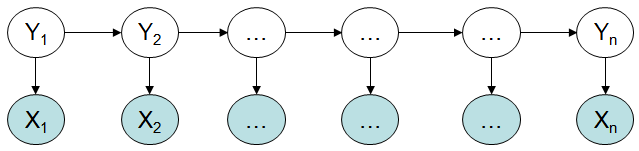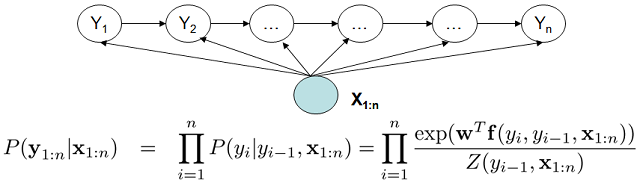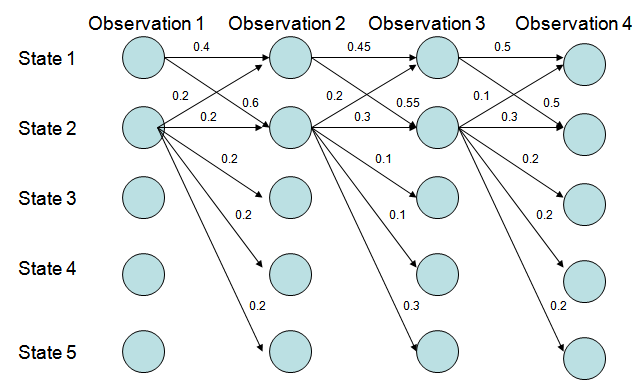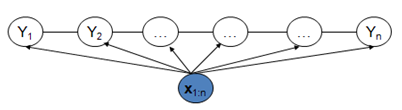# Community

Blog Events Webinars Tutorials Forum
×
Community Blog HMM, MEMM, and CRF: A Comparative Analysis of Statistical Modeling Methods

# HMM, MEMM, and CRF: A Comparative Analysis of Statistical Modeling Methods

HMM, MEMM, and CRF are three popular statistical modeling methods, often applied to pattern recognition and machine learning problems.

This article presents a comparison analysis of Hidden Markov Model (HMM), Maximum Entropy Markov Models (MEMM), and Conditional Random Fields (CRF). HMM, MEMM, and CRF are three popular statistical modeling methods, often applied to pattern recognition and machine learning problems. Let us explore each method in further detail.

### Hidden Markov Model (HMM)

The word “Hidden” symbolizes the fact that only the symbols released by the system are observable, while the user cannot view the underlying random walk between states. Many in this field recognize HMM as a finite state machine.Hidden Markov Model (HMM)

HMM has a strong statistical foundation with efficient learning algorithms where learning can take place directly from raw sequence data. It allows consistent treatment of insertion and deletion penalties in the form of locally learnable methods and can handle inputs of variable length. They are the most flexible generalization of sequence profiles. It can also perform a wide variety of operations including multiple alignment, data mining and classification, structural analysis, and pattern discovery. It is also easy to combine into libraries.

• HMM is only dependent on every state and its corresponding observed object:

The sequence labeling, in addition to having a relationship with individual words, also relates to such aspects as the observed sequence length, word context and others.

• The target function and the predicted target function do not match:
HMM acquires the joint distribution P(Y, X) of the state and the observed sequence, while in the estimation issue, we need a conditional probability P(Y|X).

### Maximum Entropy Markov Models (MEMM)Maximum Entropy Markov Models

MEMM takes into account the dependencies between neighboring states and the entire observed sequence, hence a better expression ability. MEMM does not consider P(X), which reduces the modeling workload and learns the consistency between the target function and the estimated function.

### MEMM Labeling BiasViterbi algorithm decoding of MEMM

In Figure 3, State 1 tends to convert to State 2, and State 2 tends to stay at State 2 at the same time.

P(1-> 1-> 1-> 1)= 0.4 x 0.45 x 0.5 = 0.09, P(2->2->2->2)= 0.2 X 0.3 X 0.3 = 0.018,

P(1->2->1->2)= 0.6 X 0.2 X 0.5 = 0.06,P(1->1->2->2)= 0.4 X 0.55 X 0.3 = 0.066.

However, the optimal state conversion path is 1 > 1 > 1 >1. Why?

It is because State 2 has more convertible states than State 1 does, hence reducing the conversion probability – MEMM tends to select the state with fewer convertible states. Such selection is termed the labeling bias issue. CRF well addresses the labeling bias issue.

### Conditional Random Fields (CRF model)The CRF model has addressed the labeling bias issue and eliminated two unreasonable hypotheses in HMM. Of course, the model has also become more complicated.

On the other hand, MEMMs cannot find the corresponding parameters to meet the following distribution:

a b c --> a/A b/B c/C              p(A B C | a b c) = 1
a b e --> a/A b/D e/E             p(A D E | a b e) = 1
p(A|a)p(B|b,A)p(C|c,B) = 1
p(A|a)p(D|b,A)p(E|e,D) = 1
But CRFs can.

Generative model or discriminative model

Suppose o is the observed value, and m is the model.

a) Generative model: Infinite samples > Probability density model = Generative model > Prediction

If you model P(o|m), it is a generative model. The basic idea is, first, to establish the probability density model of the sample, and then use the model for inference prediction. The requirement of the samples being infinite or as large as possible is common knowledge. The method draws from statistical mechanics and Bayes theory.

HMM directly models the transition probability and phenotype probability, and calculates the probability of co-occurrence. Thus, it is a generative model.

b) Discriminative model: Finite samples > Discriminative function = Discriminative model > Prediction

If you model on the conditional probability P(m|o), it is the discriminative model. The basic idea is to establish the discriminant function with finite samples, and directly study the prediction model without considering the generative model of samples. Its representative theory is the statistical learning theory.

CRF is a discriminant model. MEMM is not a generative model, but a model with finite states based on state classification.

Topological structure

HMM and MEMM are a directed graph, while CRF is an undirected graph.

Global optimum or local optimum

HMM directly models the transition probability and the phenotype probability, and calculates the probability of co-occurrence.

MEMM establishes the probability of co-occurrence based on the transition probability and the phenotype probability. It calculates the conditional probability, and only adopts the local variance normalization, making it easy to fall into a local optimum.

CRF calculates the normalization probability in the global scope, rather than in the local scope as is the case with MEMM. It is an optimal global solution and resolves the labeling bias issue in MEMM.

• Compared with HMM: Since CRF does not have as strict independence assumptions as HMM does, it can accommodate any context information. Its feature design is flexible (the same as ME).
• Compared with MEMM: Since CRF computes the conditional probability of global optimal output nodes, it overcomes the drawbacks of label bias in MEMM.
• Compared with ME: CRF computes the joint probability distribution of the entire label sequence when an observation sequence intended for labeling is available, rather than defining the state distribution of the next state under the current state conditions given.

CRF is highly computationally complex at the training stage of the algorithm. It makes it very difficult to re-train the model when newer data becomes available.

### Conclusion:

This post detailed out a comparative analysis between Hidden Markov Model (HMM), Maximum Entropy Markov Models (MEMM), and Conditional Random Fields (CRF). In this post we categorically learnt that CRFs and MEMMS are mainly discriminative sequence models whereas HMMs are primarily generative sequence models. It is Bayes Rule that forms the basis of HMM. On the contrary, CRF and MEMM’s based on MaxEnt models over transition and observable features.

0 0 0
Share on

# Related Products

• ##AIRec

A high-quality personalized recommendation service for your applications.

• ##Artificial Intelligence Service for Conversational Chatbots Solution

This solution provides you with Artificial Intelligence services and allows you to build AI-powered, human-like, conversational, multilingual chatbots over omnichannel to quickly respond to your customers 24/7.

• ##Big Data Consulting Services for Retail Solution

Alibaba Cloud experts provide retailers with a lightweight and customized big data consulting service to help you assess your big data maturity and plan your big data journey.

• ##Big Data Consulting for Data Technology Solution

Alibaba Cloud provides big data consulting services to help enterprises leverage advanced data technology.